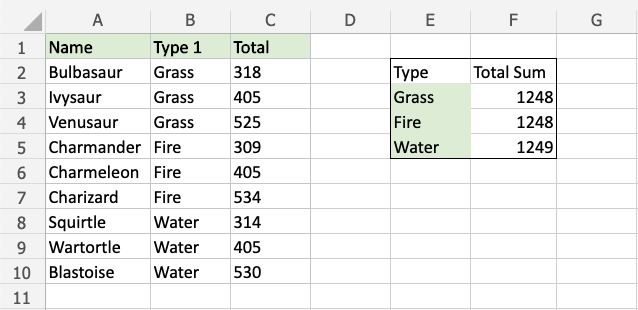# Excel SUMIF Function

## SUMIF Function

The SUMIF function is a premade function in Excel, which calculates the sum of values in a range based on a true or false condition.

It is typed `=SUMIF`:

=SUMIF(range, criteria, [sum_range])

The condition is referred to as `criteria`, which can check things like:

• If a number is greater than another number `>`
• If a number is smaller than another number `<`
• If a number or text is equal to something `=`

The `[sum_range]` is the range where the function calculates the sum.

Note: The `[sum_range]` is optional.

If not specified, the function calculates the sum of the same range as the condition.

Note: The different parts of the function are separated by a symbol, like comma `,` or semicolon `;`

The symbol depends on your Language Settings.

## Example SUMIF function

Find the sum of Total stats for Grass type Pokemon:

The condition is that the type is "Grass".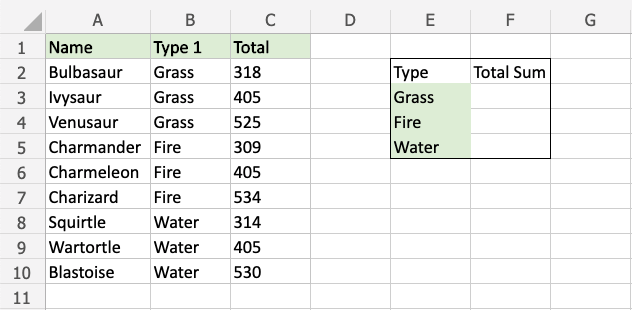Copy Values

Example SUMIF function, step by step:

1. Select the cell `F3`
2. Type `=SUMIF`
3. Double click the SUMIF command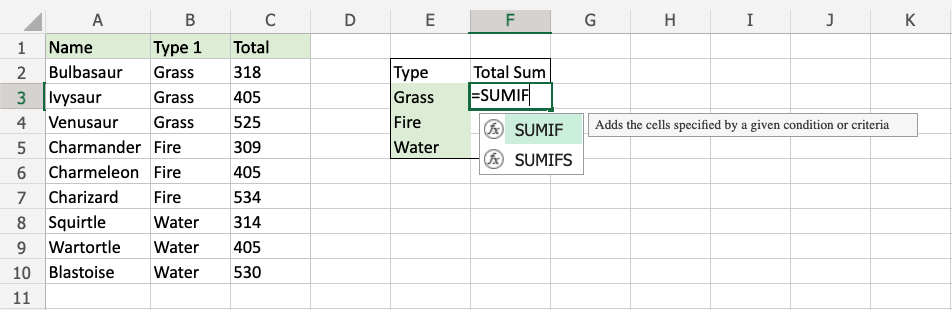1. Specify the range for the condition`B2:B10` (the Type 1 values)
2. Type `,`
3. Specify the criteria (the cell `E3`, which has the value "Grass")
4. Type `,`
5. Specify the range for the sum `C2:C10` (the Total values)
6. Type `,`
7. Hit enter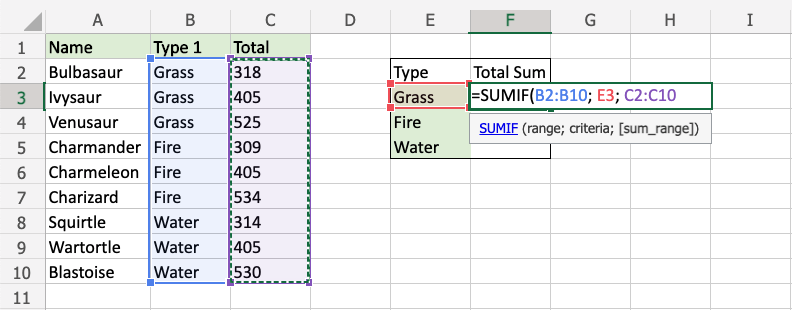The function now sums the Total stats for Grass type Pokemon.

The function can be repeated for the other Pokemon types to compare them: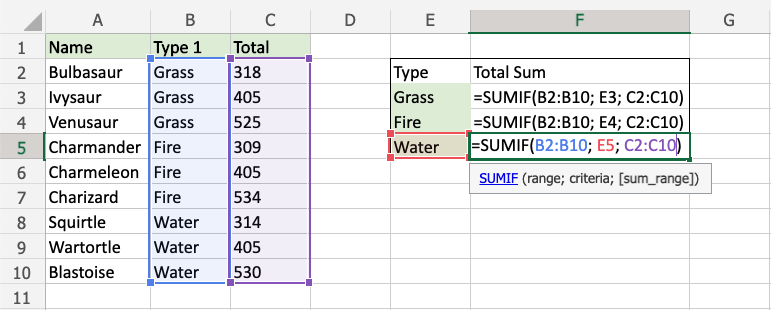Note: You can use the filling function for the other rows, but make sure to use absolute references for the ranges.

Now, we can see the sum of total stats for the different types of Pokemon: